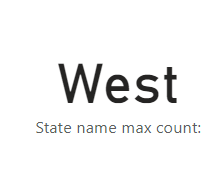cancel
Showing results for
Did you mean:Frequent Visitor

## MAXX of Category based on DistinctCount of rows

Hi,
I have a sample data below. What I'm trying to do is show the State which has the Max value of rows based on the distinct count of IDs.
Answer = West, which has 4 IDs count
I know how to show the value 4, but not the State = West.
My code:

DistinctCount = DISTINCTCOUNT(Table[ID])
Max Value = MAXX(values(Table[State]),[DistinctCount])

 ID State A West B West C East D North E West F West G East

Can anyone help me with bringing out the value "West"?

Thank you.

1 ACCEPTED SOLUTIONCommunity Support

I have checked Jihwan_Kim's code, I think his measure will alway return "West", due to it will return the max state.

My Sample:The max state in my sample should be "East".

``````State name max count: =
VAR _MaxCount =
MAXX ( VALUES ( 'Table'[State] ), [DistinctCount] )
RETURN
MAXX (
FILTER ( VALUES ( 'Table'[State] ), [DistinctCount] = _MaxCount ),
[State]
)``````

Result in my sample is as below.Test by your data, result should return "West".Best Regards,
Rico Zhou

If this post helps, then please consider Accept it as the solution to help the other members find it more quickly.

2 REPLIES 2Community Support

I have checked Jihwan_Kim's code, I think his measure will alway return "West", due to it will return the max state.

My Sample:The max state in my sample should be "East".

``````State name max count: =
VAR _MaxCount =
MAXX ( VALUES ( 'Table'[State] ), [DistinctCount] )
RETURN
MAXX (
FILTER ( VALUES ( 'Table'[State] ), [DistinctCount] = _MaxCount ),
[State]
)``````

Result in my sample is as below.Test by your data, result should return "West".Best Regards,
Rico Zhou

If this post helps, then please consider Accept it as the solution to help the other members find it more quickly.Super User

Hi,

Please check the below picture and the attached pbix file.``````State name max count: =
MAXX (
GROUPBY (
ADDCOLUMNS ( VALUES ( 'Table'[State] ), "@count", [DistinctCount] ),
'Table'[State],
"@maxcount", MAXX ( CURRENTGROUP (), [@count] )
),
'Table'[State]
)
``````

If this post helps, then please consider accepting it as the solution to help other members find it faster, and give a big thumbs up.